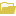# Random function

## Overview

Creates a virtual raster with random pixel values that can be used in raster function templates or mosaic datasets.

A random value is generated for every pixel in the raster.

## Notes

The Raster Info parameter includes raster dataset properties, such as the number of columns and rows, the number of bands, the pixel type, the extent, and the spatial reference. You can use the current raster information, or select a raster dataset to use as a template.

To select a raster dataset to use as a template, click the Load template raster buttonnext to the Raster Info parameter. Browse to the raster dataset you want to use and select it. This opens the Raster Information table on the dialog box, which allows you to see information about the data source you selected. You can also specify certain parameters (such as the number of bands, the cell size, the pixel depth, and the NoData value) by editing the fields enabled in the table.

While many functions work on one or more input rasters, the Random function does not require an input raster. This means that it behaves more like a raster dataset than a function, so particular care must be taken when adding it to or removing it from the function chain.

The chaining of functions takes place by using the raster created from one function as the input raster for the next one in the chain. Since the Random function requires no input raster, if it is added within a chain, everything that could have been an input to a previous function is no longer required and thus disappears. This makes it possible for your mosaic dataset to become corrupted. Safeguards are in place to prevent adding a Random function before a Mosaic function, thereby protecting the mosaic dataset. Removing the Random function requires some additional steps because of the safeguards. You cannot remove a Random function from a function chain by right-clicking the function and clicking Remove. This is because the function preceding it in the chain (the function that uses it as an input) needs to have a replacement input raster. Removing a Random function uses the same steps as removing or replacing an input raster. Right-click the function that uses the Random function as an input and click Properties. Then change the source of the input raster in the function to use a raster and not the output of the Random function.

## Parameters

Parameter nameDescription

Distribution

Specify the random value distribution method to use.

• Uniform (Minimum, Maximum)—A uniform distribution with a defined range. The default values are 0 for Minimum and 1 for Maximum. This is the default.
• Integer (Minimum, Maximum)—An integer distribution with a defined range. The default values are 1 for Minimum and 10 for Maximum.
• Normal (Mean, Standard Deviation)—A normal distribution with a defined mean and standard deviation. The default values are 0 for Mean and 1 for Standard Deviation.
• Exponential (Mean)—An exponential distribution with a defined mean. The default value is 1.
• Poisson (Mean)—A Poisson distribution with a defined mean. The default value is 1.
• Gamma (Alpha, Beta)—A gamma distribution with a defined alpha and beta. The default values are 1 for Alpha and 1 for Beta.
• Binomial (N, Probability)—A binomial distribution with a defined N and probability. The default values are 10 for N and 0.5 for Probability.
• Geometric (Probability)—A geometric distribution with a defined probability. The default value is 0.5.
• Negative Binomial (r, Probability)—A Pascal distribution with a defined r and probability. The default values are 10.0 for r and 0.5 for Probability.

Seed

The seed is an integer value and is used to initiate the random number generator. The default value is 1.

Random Number Generator

Specify the random generator algorithm that uses the seed and distribution to produce a sequence of random numbers. The available options are as follows:

• Standard C Rand
• ACM collected algorithm 599
• Mersenne Twister

The default value is Mersenne Twister.

Raster Info

Use the Load template raster button to load a template from a directory or portal.